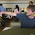## Sunday, January 3, 2016

### Sampling Arbitrary data

Introduction:
Generating data usually requires a variance - covariance matrix and is therefore restricted by using a linear assumption between the variables. However, using a linear assumption between data can miss important non - linear relationships. This post uses quantile random forests to simulate an arbitrary dataset.

MCMC Sampling:
MCMC sampling is a method that can simulate from any multivariate densities as long as one has the full conditionals. Full conditionals in this case are models of a particular variable given all other variables. For more concreteness; suppose we have a dataset with n variables (x1, x2, ... xn). The estimated full conditionals in this case are:

f(x1 | x2, x3...xn)
f(x2 | x1, x3...xn)
.
.
f(xn | x1, x3...x(n-1))

Where f() is a machine learning algorithm of choice that can provide a distribution of values, in this case I use quantregForest() for continuous variables and randomForest() for categorical variables.

Once the models are built the algorithm is as follows:
- Choose random observation as starting point
-for each iteration of N iteration
- for each variable
- Sample proposal observation from predicted distribution and compare likelihood with current observation. Accept proposal with min(p(proposal)/p(current),1)

Code can be found here.

Results:
A random forest model was built to predict whether the simulated data could be distinguished from original data. The resulting KS test (out of sample) was insignificant so we retain null that model can't distinguish between original and simulated data.

While that is a good result and shows that a model can't distinguish between simulated and original data, visually we can see a difference between the two datasets shown below.

Original Iris Data

The simulated dataset shows sharper boundaries between points that are not found in original dataset. For example, the scatterplot between Sepal.Length and Sepal.Width shows a sharp boundary at approximately 5 Sepal.Length in simulated data but it more gradual in original data.

Conclusion:
This post discussed a method to simulate an arbitrary dataset. While I cannot build a model to distinguish the two datasets, visually they are distinguishable.

***Updated with code from Dmytro Perepolkin - Thanks!

1.Hi,

Thanks for interesting post. Couple of comments and questions:

1) It might be better to illustrate classes with colors in your graphs. Replace last three lines in your code with:

gpairs(iris, scatter.pars = list(col=as.numeric(iris\$Species)))
niris <- new_data[seq(1,nrow(new_data),5)[1:150],]
gpairs(niris, scatter.pars = list(col=as.numeric(as.factor(niris\$Species))))

2) There seems to be class imbalance in your simulated data - very few 'virginica' samples. Is there a way to improve that?

3) The generated samples seem to be more "organized" than the authenitic data. Is there a way to infuse a bit more white noise into the process? Is there a way to utilize model deficiencies (i.e. FP and FN misclassification errors) to generate more "realistic" data?

1.Hey Dmytro,

1) Thanks for code! - Updated post

2) Yes there is a class imbalance. I tried rerunning it with more chains / iterations but that did alleviate the problem. My hunch is that virgnica is so distinct its hard to transition from virginica to the other two types or vice versa. That is, starting at a random data point that is virginica, if we predict what the species is given everything else, the fitted probability distribution of species will be 1 for virginica and 0 for the other two. So it might not sample correctly because of this limitation.

3) That's an interesting thought. I've just been using predicted probabilities for transitions. Maybet he algorithm could compare the predicted probabilities with out of sample prediction rates, and the difference can be an additional error term.

Thanks for comments and questions. If you have any thoughts or further input let me know!